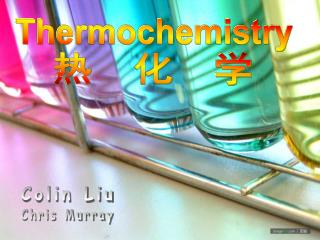DownloadDownload PresentationThermochemistry

# Thermochemistry

Download Presentation## Thermochemistry

- - - - - - - - - - - - - - - - - - - - - - - - - - - E N D - - - - - - - - - - - - - - - - - - - - - - - - - - -
##### Presentation Transcript

1. Thermochemistry 热 化 学 Colin Liu Chris Murray

2. au revoir facile !!! What is thermochemistry? Thermochemistry is the study of heat changes that occur during chemical reactions.

3. Energy 能量 Energy is the capacity for doing work or supplying heat. We can also state that the energy is the capacity to produce changes. It is found in two forms: Stored energy Potential Kinetic Energyofmotion

4. Heat 热 Heat is a form of energy Heat and temperature are not the same. Heat involves a transfer ofenergy between two objects because of a difference in temperature. Temperature is the measureof the average kinetic energy of molecules. Any temperature change (ΔT)depends on the amountof heat (q) flowing into or out of the substance.

5. Conservation of Energy 能量守恒定律 No energy is created or destroyed as a result of these heats of transfers.

6. What do u think of my dancing Exothermic 吸热: A process that releases heat that flows to the surroundings Endothermic 放热: A process that absorbs heat from the surroundings.

7. Calorimetry 量热法 A calorimeter is used to determine the heat released by a reaction. In calorimetry, the heat evolves by the system is equal to the heat absorbed by the surroundings. Calorimeters are devices used to measure the absorptions or release of heat in physical or chemical processes.

8. Enthalpy 焓 Enthalpy (H) is the amount of heat that a substance has at a given temperature and pressure. Heat and enthalpy are the same for reactions at constant pressure.

9. Molar Enthalpies for phase changes 相变单位焓 Molar Enthalpy of Fusion (Hfus) – is the heat absorbed by one mole of a substance when melting from a solid to a liquid at the same temperature. Molar enthalpy of freezing (Hfr) is the same amount of heat released when the substance solidifies from a liquid to as solid.

10. Thermochemical Equations 热化学方程式 A thermochemical equation includes the heat change. Ex)CaO(s) + H2O(l)  Ca(OH)2(s) + 65.2 kJ

11. This is a good picture of Mr.T Last thing LOL